# Math: Elementary School: 1st and 2nd Grade Quiz - Describe 2D Shapes (Questions)

This Math quiz is called 'Describe 2D Shapes' and it has been written by teachers to help you if you are studying the subject at elementary school. Playing educational quizzes is a fun way to learn if you are in the 1st or 2nd grade - aged 6 to 8.

It costs only \$12.50 per month to play this quiz and over 3,500 others that help you with your school work. You can subscribe on the page at Join Us

When talking about 2-dimensional shapes and other related objects, elementary school children will understand that they can use different words to describe their properties. They will use language such as sides, edges, corners and faces and recognize that some shapes have the same name but may look different.

This quiz will familiarize your child with the language used to describe 2-D shapes so that they can accurately name them and other related objects.

Can you recognize the properties of these 2D shapes?

Click on the pictures for a closer look.

1. This little girl is holding...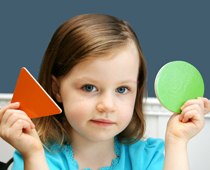[ ] a triangle and a circle [ ] a triangle and a square [ ] a circle and a rectangle [ ] a rectangle and a square
2. What shape is this clock face?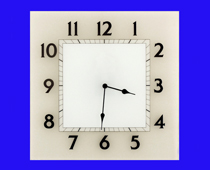[ ] A rectangle [ ] A circle [ ] A square [ ] A triangle
3. What shape are the pairs of triangles making?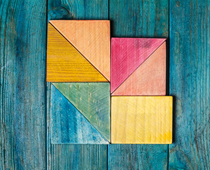[ ] Oblongs [ ] Circles [ ] Triangles [ ] Squares
4. This triangle is called an equilateral triangle because all its sides and angles are..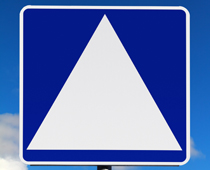[ ] different lengths [ ] the same color [ ] made of plastic [ ] the same
5. The colored part of the eye is a...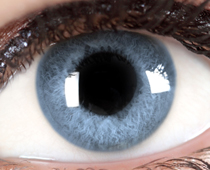[ ] rectangle [ ] square [ ] triangle [ ] circle
6. How are rectangles different to squares?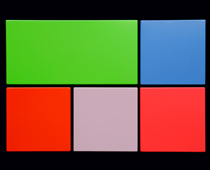[ ] They don't have as many corners [ ] They have 4 sides of different lengths [ ] They have two long and two short sides [ ] They have more sides
7. This pattern is made up from rectangles. Which shape wouldn't fit together as well?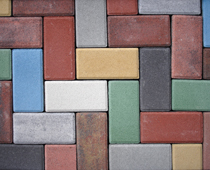[ ] Triangles [ ] Circles [ ] Squares [ ] Cubes
8. Which shape has the most corners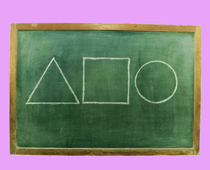[ ] They all have the same number of corners [ ] The circle [ ] The triangle [ ] The square
9. A square is a kind of...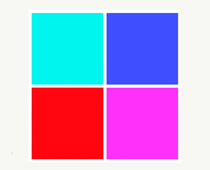[ ] sphere [ ] triangle [ ] circle [ ] rectangle
10. This road sign is a...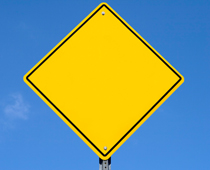[ ] triangle [ ] square [ ] oval [ ] oblongMath: Elementary School: 1st and 2nd Grade Quiz - Describe 2D Shapes (Answers)
1. This little girl is holding...[x] a triangle and a circle [ ] a triangle and a square [ ] a circle and a rectangle [ ] a rectangle and a square
A triangle has three straight sides and a circle has one curved side
2. What shape is this clock face?[ ] A rectangle [ ] A circle [x] A square [ ] A triangle
All four sides are the same length
3. What shape are the pairs of triangles making?[ ] Oblongs [ ] Circles [ ] Triangles [x] Squares
Two right triangles pushed together can make a square
4. This triangle is called an equilateral triangle because all its sides and angles are..[ ] different lengths [ ] the same color [ ] made of plastic [x] the same
Equilateral means equal or the same
5. The colored part of the eye is a...[ ] rectangle [ ] square [ ] triangle [x] circle
The iris and pupil are both circles
6. How are rectangles different to squares?[ ] They don't have as many corners [ ] They have 4 sides of different lengths [x] They have two long and two short sides [ ] They have more sides
Squares have 4 sides of equal length
7. This pattern is made up from rectangles. Which shape wouldn't fit together as well?[ ] Triangles [x] Circles [ ] Squares [ ] Cubes
Because circles are curved, they don't fit together at all!
8. Which shape has the most corners[ ] They all have the same number of corners [ ] The circle [ ] The triangle [x] The square
The square has four corners, the triangle has three and the circle has no corners
9. A square is a kind of...[ ] sphere [ ] triangle [ ] circle [x] rectangle
A rectangle has four sides - a square is a kind of rectangle with four equal sides
10. This road sign is a...[ ] triangle [x] square [ ] oval [ ] oblong
Even though it is tilted, the shape is still a square